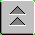[Index]

Frequency Schedules

HF (3-30 MHz) radio waves propagate either as line-of-sight (space) or sky waves. Sky waves are the most useful to understand because they provide the longest geometric range possible (independant of transmitted power). The reason is simple: assuming propagation in a straight line, if the signal reflects off something at a significant altitude the maximum range is extended. Usually the straight line distance is limited by the horizon (the point where the straight line path is tangent to the earth). A sky wave can extend the propagation path well beyond the visible horizon.

The higher the reflecting height, H, the greater the range (for a given angle of incidence).

Key facts:

-the reflecting layer height goes up with the Sun (and back down), reaching a maximum at local noon.

-the ionosphere absorbs radio waves, having maximum absorption at the lower frequencies and less as the frequency increases

-frequencies above a maximum value are not reflected at all and continue into space.

Conclusions:

-Use the maximum frequency that will reflect (called maximum useable frequency, MUF)

-As the layer height increases, the MUF also increases.

Frequency schedule: changes the frequency in use during the day to use the highest frequency that will reflect. So highest frequencies are at local noon, lowest at night.